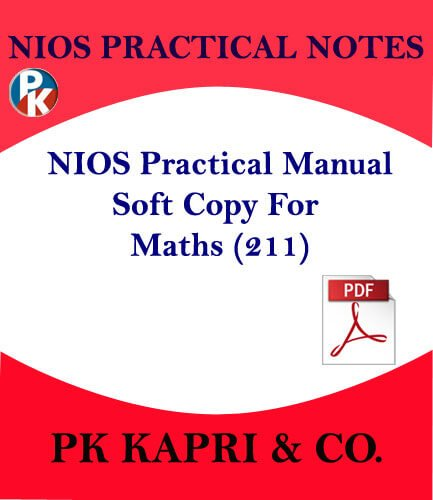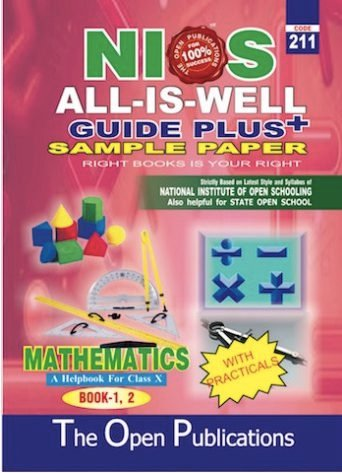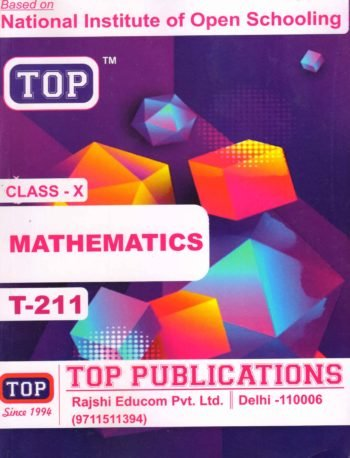Hot
-70%

## 211 NIOS PRACTICAL MANUAL MATHEMATICS 211 NOTES IN ENGLISH MEDIUM -PDF

0 out of 5

200.00 60.00

211 NIOS PRACTICAL MANUAL MATHEMATICS 211 NOTES IN ENGLISH MEDIUM -PDF

### Frequently Bought Together++Price for all:   570.00
• Description

## Description

211 NIOS PRACTICAL MANUAL MATHEMATICS 211 NOTES IN ENGLISH MEDIUM -PDF

Here We Provide NIOS Practical Lab Manual &amp; Practical Examination Guidelines for 10th Class with important Experiments, Marking Scheme.

Guidelines for Nios (211) Mathematics Practical Examination Secondary Level

There will be a practical examination of 15 marks apart from the theory examination.

The distribution of 15 marks is as follows:

Assessment of Activity Performed/Records of activities prepared                         10 Marks

Viva – voce                                                                                                                           5 Marks

(i) The proposed practical test is suggested to be held at least 15 days before the theory
examination.
(ii) Every students may be given two activities out of which he has to select one and
perform it these (in case, the students not comfortable with the given activities, he
may be allowed to select one activity of his choice)
(iii) Viva-voce can be done at the examination centre by asking questions related to the
activity/project he/she has done.

List of Practical works :

• Verification of the identity (a+b)2 = a2 + 2ab + b2
• Verification of the identity (a-b) 2 = a2 – 2ab + b2
• Verification of the identity (a2-b2) = (a +b) (a-b)
• Verification of the identity (a+b)3 = a3 + b3 +3a2b+3ab2
• To find the HCF of two given numbers by division method.
• Equivalent fractions
• To verify that a linear equation in two variables has infinite number of solutions.
• To find the condition for consistency of a system of linear equations.
• To verify the relation between roots and coefficients of a quadratic equation.
• To verify graphically that a quadratic polynomial can have at most two zeroes.
• To verify that a given sequence is an A.P.
• To find the sum of first n odd natural numbers.
• To find the sum of first n natural numbers.
• To find the sum of first n terms of an arithmetic progression.
• To verify that the sum of the angles of a triangle is 180o
• To verify that the angles opposite to equal sides of a triangle are equal.
• To verify mid point theorem.
• To verify basic proportionality theorem.
• To verify Pythagoras theorem.
• To verify the relation between the ratio of areas of two similar triangles and their sides.
• To find the area of a circle.
• To demonstrate that the opposite angles of a cyclic quadrilateral are supplementary.
• To verify that the equal chords of congruent circles subtend equal angles at the centre.
• To find the area of a trapezium.
• To find the total surface area of a cube.
• To find the formula for the curved surface area of a cone.
• To find the relationship among the volumes of right circular cone, right circular cylinder and a hemisphere of same radius and same height.
• To verify the identity a3 − b3 = (a − b)(a2 + ab + b2 )
• To draw a triangle equal in area to a parallelogram.
• To find the incentre of different types of triangles.
• This PDF is meant to tell you all about all the sort of work which you all have to keep In mind while making a practical file of 10th / 12th and my purpose is only to make your work easy. This PDF is strictly based on the NIOS Class Xth /XIIth Practical File in Hindi Medium or English Medium and includes only important content and guidelines prescribed for students of 10th or 10th +2 level.
• Declaration/Note.
1. The Goods/Product sold are end user consumption and not for resale.
2. The is only reference material for lab manual, this is not solved practical manual.
2. This is digital product this is not refundable or changeable please read all the details carefully before buying.
error: Content is protected !!